# GRE Subject Test: Biochemistry, Cell, and Molecular Biology : Help with Acid-Base Chemistry and pH

## Example Questions

← Previous 1

### Example Question #1 : Help With Acid Base Chemistry And P H

What is the appropriate way to describe a solution with a pH of 3 compared to a solution with a pH of 6?

It is 30 times more acidic

It is 3 times less acidic

It is 3 times more acidic

It is 1000 times more acidic

It is 1000 times more acidic

Explanation:

The pH scale is logarithmic, meaning every whole number change on the scale reflects a ten-fold change in acidity. Since a pH of 3 is three numbers higher than a pH of 6, we can find the change in acidity by taking 10 to the third power.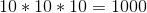The solution with a pH of 3 is 1000 times more acidic than the solution with a pH of 6.

### Example Question #1 : Help With Acid Base Chemistry And P H

What is the pH of a 0.03M hydrochloric acid solution?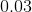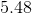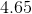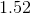Explanation:

Since hydrochloric acid is considered a strong acid, we can assume that the acid will dissociate completely, leaving us with a 0.03M concentration of hydronium ions. Knowing this, we can calculate the pH by taking the negative log of the hydronium concentration: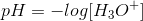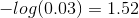### Example Question #6 : Chemical Reactions

What is the concentration of hydroxide ions in a 1M hydrochloric acid solution?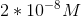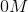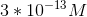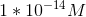Explanation:

To solve this question we need to first look at the relationship between hydroxide and hydrogen ion concentrations: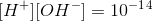where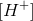is concentration of hydrogen ions and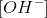is the concentration of hydroxide ions. Solve for: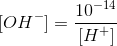We can solve for the concentration of hydrogen ions in this solution by writing the dissociation of hydrochloric acid in solution: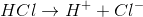The question states that it is a 1M solution. Since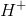and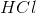are in 1:1 ratio, the concentration of hydrogen ions will equal the concentration; therefore,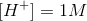. Note that this won’t be the case if the acid was weak. For a weak acid, the concentration ofwill be less than the concentration of acid, even if it was 1:1 ratio. This occurs because a weak acid does not dissociate completely.

We can now use theto solve for.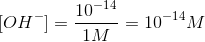Therefore, concentration of hydroxide ions in this solution is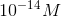### Example Question #7 : Chemical Reactions

Which of the following substances is considered a Lewis acid?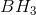Both of these compounds

None of these compounds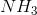Explanation:

Recall that acids and bases can be defined in three different ways. First, the Arrhenius definition states that an acid is a substance that increases the concentration of hydrogen ions in solution whereas a base decreases hydrogen ions concentration. Second, Bronsted-Lowry definition states that an acid is any substance that donates a proton whereas a base is any substance that accepts a proton. Finally, Lewis definition states that an acid is any substance that accepts an electron pair whereas a base is a substance that donates an electron pair.

We have two substances in this question., or borane, is considered a Lewis acid because it accepts an electron pair. Boron has four orbitals in its outermost shell. If we look at Boron in borane, we will notice that three orbitals are occupied by the electrons in the three single bonds (bound to hydrogen) whereas the last orbital is empty and is free to accept electrons. Since it accepts electrons, borane is considered a lewis acid., or ammonia, is a lewis base. If we look at the nitrogen atom in ammonia, we will notice that all of its orbitals are occupied (three orbitals are occupied with the three bonds to hydrogen atoms and the last orbital is occupied with a lone electron pair). Since it has extra electrons in the last orbital, ammonia can donate electrons and is considered a lewis base.

### Example Question #8 : Chemical Reactions

Solutions A and B are both made up of an acidic solution. After analysis, it is observed that the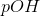of solution A is higher whereas the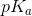of acid in solution B is lower. What can be concluded about these results?

More products are produced in solution A

More hydrogen ions are produced in solution B

Results seem invalid

The acid dissociation constant of solution A is higher

Results seem invalid

Explanation:

The question states that theof solution A is higher. The definition ofis: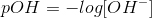If we rearrange and solve for concentration of hydroxide ions, we get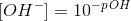This means thatdecreases asincreases. The relationship between hydroxide ion and hydrogen ion is as follows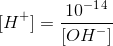Note that the hydroxide ion concentration is inversely proportional to that of hydrogen ions; therefore, lower hydroxide ion concentration leads to higher hydrogen ion concentration and higher acidity. Since solution A has the higher, its hydroxide ion concentration is lower than solution B. This means that solution A has higher hydrogen ion concentration and, therefore, is more acidic than solution B.

The question also states that the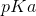of solution B is lower. Recall that asof an acid decreases it becomes more acidic. Based on this, we conclude that solution B is more acidic. The two results given in this question conflict each other; therefore, these results seem invalid.

### Example Question #9 : Chemical Reactions

The pH of a solution __________ when the acid dissociation constant __________.

does not change . . . increases

increases . . . decreases

does not change . . . decreases

increases . . . increases

increases . . . decreases

Explanation:

Acid dissociation constant, or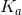, for a prototypical acid-base reaction is defined as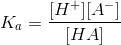where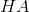is an acid and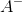is its conjugate base. Based on this equation, we can determine thatandare directly proportional to one another; therefore, increasingwill increaseand vice versa.

Recall the definition of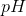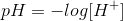Solving forgives us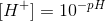This means that increasing pH will decreaseand vice versa (has the opposite effect on each other); therefore,andalso have an opposite effect on each other.

### Example Question #91 : Gre Subject Test: Biochemistry, Cell, And Molecular Biology

A buffer can be created by adding equal amounts of weak acid and its conjugate base. What additional information is needed to determine the pKa of a buffer made in this manner?

None of these are needed

Both of these are needed

pH

Concentration of acid and conjugate base

pH

Explanation:

The buffer is made by using weak acid and its conjugate base. The relationship between the concentration of the components (acid and conjugate base), pKa, and pH can be determined by using the Henderson-Hasselbalch equation.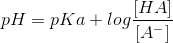whereis weak acid andis the conjugate base. We know that equal amounts ofandare added; therefore,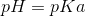for buffer solutions made in this manner. To calculate the pKa of the solution we need the pH.

### Example Question #91 : Gre Subject Test: Biochemistry, Cell, And Molecular Biology

A researcher adds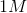acid to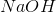. The concentration of hydrogen ions is __________ as the concentration of hydroxide ions.

three times as much

half as much

the same

twice as much

the same

Explanation:

Hydrochloric acid () and sodium hydroxide () are both strong reagents. Adding equal amounts of strong acid to a strong base neutralizes the solution and gives the solution a neutral pH of 7. This means that the concentration of hydrogen ions and hydroxide ions are equal to each other. If pH was greater than 7 (basic pH), then the hydroxide ion concentration would be higher and if pH was less than 7 (acidic pH) then hydrogen ion concentration would be higher.

### Example Question #3 : Help With Acid Base Chemistry And P H

Solution A contains a weak acid and a weak base whereas solution B contains a weak acid and a strong base. What can you conclude about these two solutions?

I. Solution B will have a higher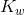II. Solution B will have a higher pOH

III. Solution A will have a lower concentration of hydroxide ions

III only

I and II

None of these

II and III

III only

Explanation:

Solution A has two weak reagents whereas solution B contains a strong base. This means that solution B will be more basic, will have a higher concentration of hydroxide ions, and a higher pH.is a constant and is not altered by the relative reagents (it is always equal to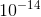). Recall that pOH is defined asThis equation suggests that pOH decreases as the hydroxide ion concentration increases. Since it has the higher hydroxide ion concentration, solution B will have a lower pOH.

### Example Question #4 : Help With Acid Base Chemistry And P H

What is the pH of a solution composed of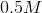acetic acid and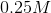acetate?for acetic acid is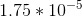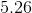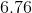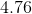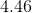Explanation:

Since this problem involves both the acid and the conjugate base of the acid, we can use the Henderson-Hasselbach equation in order to determine the pH of the solution: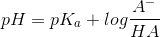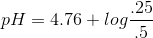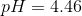← Previous 1

### All GRE Subject Test: Biochemistry, Cell, and Molecular Biology Resources